OpenCV  4.4.0 Open Source Computer Vision
Meanshift and Camshift

Prev Tutorial: How to Use Background Subtraction Methods

Next Tutorial: Optical Flow

## Goal

In this chapter,

• We will learn about the Meanshift and Camshift algorithms to track objects in videos.

## Meanshift

The intuition behind the meanshift is simple. Consider you have a set of points. (It can be a pixel distribution like histogram backprojection). You are given a small window (may be a circle) and you have to move that window to the area of maximum pixel density (or maximum number of points). It is illustrated in the simple image given below: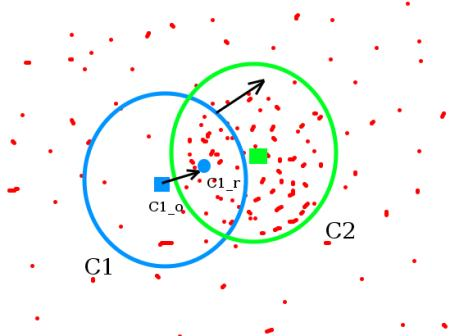image

The initial window is shown in blue circle with the name "C1". Its original center is marked in blue rectangle, named "C1_o". But if you find the centroid of the points inside that window, you will get the point "C1_r" (marked in small blue circle) which is the real centroid of the window. Surely they don't match. So move your window such that the circle of the new window matches with the previous centroid. Again find the new centroid. Most probably, it won't match. So move it again, and continue the iterations such that the center of window and its centroid falls on the same location (or within a small desired error). So finally what you obtain is a window with maximum pixel distribution. It is marked with a green circle, named "C2". As you can see in the image, it has maximum number of points. The whole process is demonstrated on a static image below: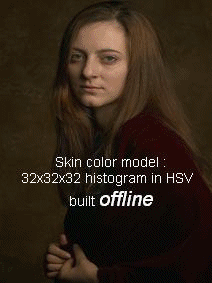image

So we normally pass the histogram backprojected image and initial target location. When the object moves, obviously the movement is reflected in the histogram backprojected image. As a result, the meanshift algorithm moves our window to the new location with maximum density.

### Meanshift in OpenCV

To use meanshift in OpenCV, first we need to setup the target, find its histogram so that we can backproject the target on each frame for calculation of meanshift. We also need to provide an initial location of window. For histogram, only Hue is considered here. Also, to avoid false values due to low light, low light values are discarded using cv.inRange() function.

Three frames in a video I used is given below: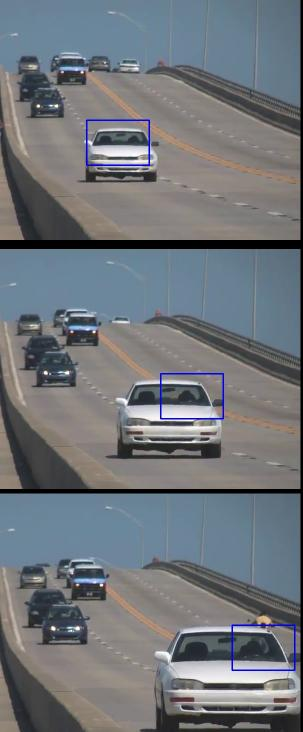image

## Camshift

Did you closely watch the last result? There is a problem. Our window always has the same size whether the car is very far or very close to the camera. That is not good. We need to adapt the window size with size and rotation of the target. Once again, the solution came from "OpenCV Labs" and it is called CAMshift (Continuously Adaptive Meanshift) published by Gary Bradsky in his paper "Computer Vision Face Tracking for Use in a Perceptual User Interface" in 1998  .

It applies meanshift first. Once meanshift converges, it updates the size of the window as, $$s = 2 \times \sqrt{\frac{M_{00}}{256}}$$. It also calculates the orientation of the best fitting ellipse to it. Again it applies the meanshift with new scaled search window and previous window location. The process continues until the required accuracy is met.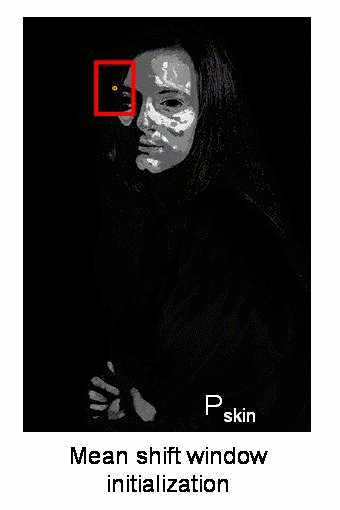image

### Camshift in OpenCV

It is similar to meanshift, but returns a rotated rectangle (that is our result) and box parameters (used to be passed as search window in next iteration). See the code below:

Three frames of the result is shown below: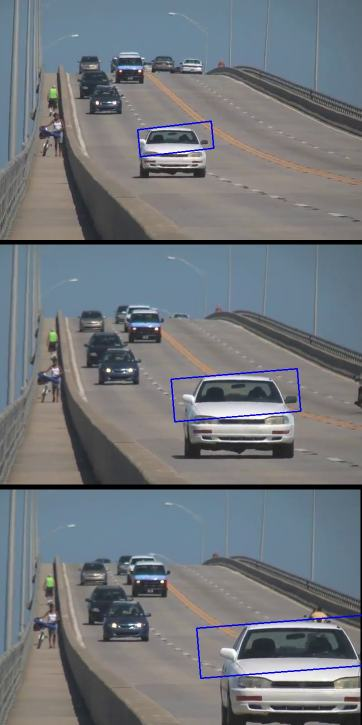image